# Writing Numbers in Scientific Notation

Author: Sophia Tutorial
##### Description:

Write numbers in scientific notation as numbers in standard form, or vice versa.

(more)

Sophia’s self-paced online courses are a great way to save time and money as you earn credits eligible for transfer to many different colleges and universities.*

No credit card required

37 Sophia partners guarantee credit transfer.

299 Institutions have accepted or given pre-approval for credit transfer.

* The American Council on Education's College Credit Recommendation Service (ACE Credit®) has evaluated and recommended college credit for 33 of Sophia’s online courses. Many different colleges and universities consider ACE CREDIT recommendations in determining the applicability to their course and degree programs.

Tutorial
what's covered
1. Writing Numbers in Scientific Notation

# 1. Writing Numbers in Scientific Notation

One application of exponent properties comes from scientific notation. Scientific notation is used to represent really large or really small numbers. An example of really large numbers would be the distance that light travels in a year measured in miles. An example of really small numbers would be the mass of a single hydrogen atom in grams. Doing basic operations such as multiplication and division with these numbers would normally be very cumbersome. However, our exponent properties make this process much simpler.

First we will take a look at what scientific notation is. Scientific notation has two parts: a number between one and ten (it can be equal to one, but not ten), and a power of ten (10 raised to an exponent power).

Scientific Notation: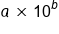where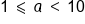The exponent, b, is very important to how we convert between scientific notation and normal numbers, or standard notation. The exponent tells us how many times we will multiply by a factor of 10. Multiplying by 10 in effect moves the decimal point one place. So the exponent will tell us how many times the exponent moves between scientific notation and standard notation. To decide which direction to move the decimal (left or right) we simply need to remember that positive exponents mean in standard notation we have a big number (bigger than ten) and negative exponents mean in standard notation we have a small number (less than one).

Keeping this in mind, we can easily make conversions between standard notation and scientific notation.

 Convert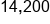to scientific notation Put decimal after first nonzero number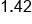Exponent is how many times decimal moved,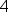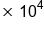Positive exponent, standard notation is big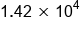Our Solution Convert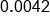to scientific notation Put decimal after first nonzero number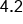Exponent is how many times decimal moved,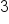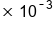Negative exponent, standard notation is small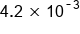Our Solution

big idea
When converting into scientific notation, if we move the decimal to the left, this increases the exponent. If we move the decimal to the right, this decreases the exponent.
hint
Recall that the decimal number in scientific notation must be at least 1, but no greater than 10. This means that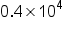and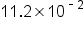are not in proper scientific notation. To correct these types of expressions, the decimal needs to shift either to the right or to the left, to fit our rules for what the decimal number can be:
•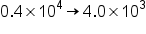: 0.4 needs to be written as 4.0, and the exponent needs to change from 4 to 3 (decreasing due to a shift to the right).
•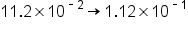: 11.2 needs to be written as 1.12, and the exponent needs to change from -2 to -1 (increasing due to a shift to the left).

We can use similar thinking to convert from a number written in scientific notation into standard notation. For these types of conversions, remember that a positive exponent means a large number, and a negative exponent means a small number.

 Convert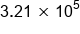to standard notation Positive exponent means standard notation big number. Move decimal right 5 places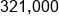Our Solution Convert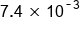to standard notation Negative exponent means standard notation is a small number. Move decimal left 3 places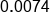Our Solution

did you know
Archimedes (287 BC - 212 BC), the Greek mathematician, developed a system for representing large numbers using a system very similar to scientific notation. He used his system to calculate the number of grains of sand it would take to fill the universe. His conclusion was 1063 grains of sand because he figured the universe to have a diameter of 1014 stadia or about 2 light years.

term to know
Scientific Notation
A way to express numbers as the product of a decimal number and a power of 10.

summary
It is important to remember that when you're writing numbers in scientific notation, you can only have one non-zero digit to the left of the decimal, but you can have any number of digits to the right. Also keep in mind when you're writing in scientific notation, moving the decimal to the left is going to increase your exponent and moving the decimal to the right is going to decrease your exponent. When you're writing in standard form, a positive exponent indicates moving the decimal to the right and a negative exponent indicates moving the decimal to the left.

Terms to Know
Scientific Notation

A way to express numbers as the product of a decimal number and a power of 10.

Rating